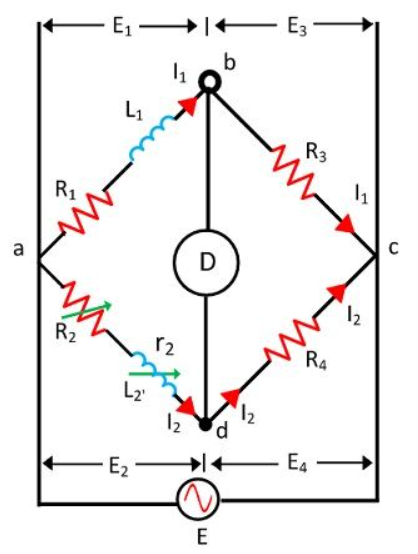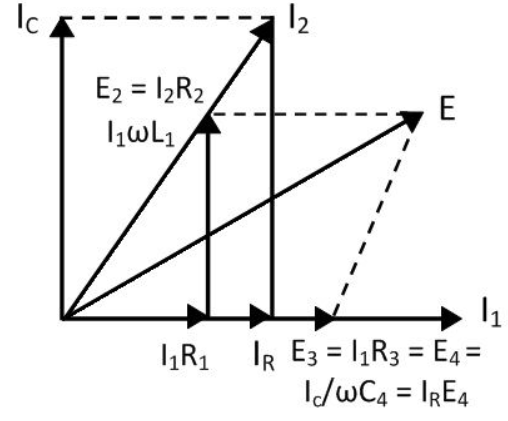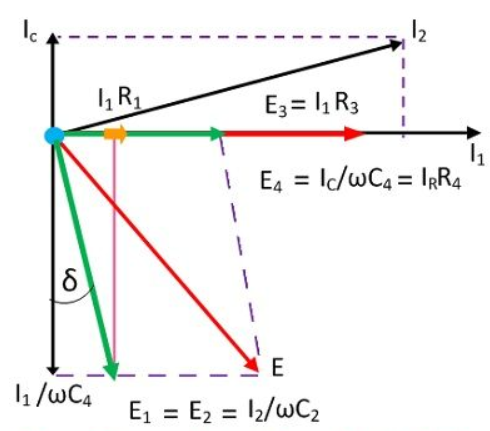### Inductance Measurement

Two methods are used for determining the self-inductance of the circuit. They are

1. Maxwell’s Inductance Bridge

2. Maxwell’s inductance Capacitance Bridge

## Maxwell’s Inductance Bridge

• A Maxwell bridge is a modification to a wheatstone bridge used to measure an unknown inductance(usually of low Q value) in terms of calibrated resistance and inductance or resistance and capacitance. When the calibrated components are a parallel resistor and capacitor, the bridge is known as a Maxwell-Wien bridge.

• It uses the principle that the positive phase angle of an inductive impedance can be compensated by the negative phase angle of a capacitive impedance when put in the opposite arm and the circuit is at resonance; i.e., no potential difference across the detector and hence no current flowing through it. The unknown inductance then becomes known in terms of this capacitance.

• With reference to the picture,Let, L1 – unknown inductance of resistance R1.

L2 – Variable inductance of fixed resistance r1.

R2 – variable resistance connected in series with inductor L2.

R3, R4 – known non-inductance resistance

At balance,

• The value of the R3 and the R4 resistance varies from 10 to 1000 ohms with the help of the resistance box. Sometimes for balancing the bridge, the additional resistance is also inserted into the circuit.

• The phasor diagram of Maxwell’s inductance bridge is shown in the figure below.## Maxwell’s Inductance Capacitance Bridge

• In this type of bridges, the unknown resistance is measured with the help of the standard variable capacitance. The connection diagram of the Maxwell Bridge is shown in the figure below.• Let, L1 – unknown inductance of resistance R1.

• R1 – Variable inductance of fixed resistance r1.

• R2, R3, R4 – variable resistance connected in series with inductor L2.

• C4 – known non-inductance resistance

• For balance condition,

• By separating the real and imaginary equation we get,

• The above equation shows that the bridges have two variables R4 and C4 which appear in one of the two equations and hence both the equations are independent.

• The circuit quality factor is expressed as

• Phasor diagram of Maxwell’s inductance capacitance bridge is shown in the figure below.

• Phasor Diagram## Schering Bridge

The Schering bridge use for measuring the capacitance of the capacitor, dissipation factor, properties of an insulator, capacitor bushing, insulating oil and other insulating materials. It is one of the most commonly used AC bridge. The Schering bridge works on the principle of balancing the load on its arm.

Let, C1 – capacitor whose capacitance is to be determined,

r1 – a series resistance, representing the loss of the capacitor C1.

C2 – a standard capacitor (The term standard capacitor means the capacitor is free from loss)

R3 – a non-inductive resistance

C4 – a variable capacitor.

R4 – a variable non-inductive resistance parallel with variable capacitor C4.

When the bridge is in the balanced condition, zero current passes through the detector, which shows that the potential across the detector is zero. At balance condition

Z1/Z2 = Z3/Z4

Z1Z4 = Z2Z3

So,

Equating the real and imaginary equations, we get

The equation (1) and (2) are the balanced equation, and it is free from the frequency.

The dissipation factor obtains with the help of the phasor diagram. The dissipation factor determines the rate of loss of energy that occurs because of the oscillations of the electrical and mechanical instrument.By the help of the above equation, we can calculate the value of tanδ which is the dissipation factor of the Schering bridge.